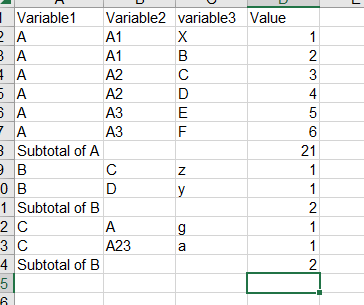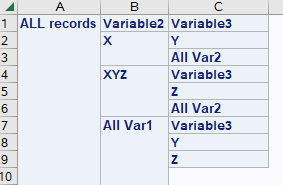## Proc tabulate, subtotal with multiple levels

Hello,

Is it possible to create a subtotal when using proc tabulate and having more than two variables.  I want the result showed in the picture below.

My code that im working with is the following:

PROC TABULATE DATA=my_df;
CLASS  Variable1 Variable2 Variable3;
VAR Response_variable;
TABLE  (Variable1 all) * ( Variable2 * Variable3),  Response_variable*(SUM=' ');
RUN;

However that code doesn't provide the result i want, once again i want it on the format that can be seen in the picture below.1 ACCEPTED SOLUTION

Accepted Solutions

## Re: Proc tabulate, subtotal with multiple levels

@FilipAxelsson wrote:

When im using that code i get the following result. Which doesnt calculate a subtotal, the only column that has a subtotal is C. Is there another way to create a crosstab with subtotals in the way i want it? (Picture in the first post)The code was to show where the ALL goes with a label that shows in the output and how the nesting of Tabulate works.

If you nest a variable to the right of an ALL you get those subgroups, you don't get to "skip" them. That is not a tabulate feature.

What you want is likely not going to come from Tabulate.

Consider this Proc Report though (and notice that I use a SAS data set that you have so can actually test code SASHELP.CARS is one of the sets SAS supplies for training in your install).

```proc report data=sashelp.cars spanrows;
where make in ('Acura' 'Audi' 'BMW');
columns make type cylinders mpg_city;
define make /group;
define type/group ;
define cylinders/group;
define mpg_city/ mean;
break after make/summarize;
run;```
4 REPLIES 4

## Re: Proc tabulate, subtotal with multiple levels

I want to clearify that Variable1 has more than 1 value## Re: Proc tabulate, subtotal with multiple levels

The general rule for "All" with subgroups is to use it with the lower level. See the result  of running the code below.

```PROC TABULATE DATA=my_df;
CLASS  Variable1 Variable2 Variable3;
VAR Response_variable;
TABLE  (Variable1 all='ALL records') * ( Variable2 All='All Var1') * (Variable3 all='All Var2'),  Response_variable*(SUM=' ');
RUN;

```

## Re: Proc tabulate, subtotal with multiple levels

When im using that code i get the following result. Which doesnt calculate a subtotal, the only column that has a subtotal is C. Is there another way to create a crosstab with subtotals in the way i want it? (Picture in the first post)## Re: Proc tabulate, subtotal with multiple levels

@FilipAxelsson wrote:

When im using that code i get the following result. Which doesnt calculate a subtotal, the only column that has a subtotal is C. Is there another way to create a crosstab with subtotals in the way i want it? (Picture in the first post)The code was to show where the ALL goes with a label that shows in the output and how the nesting of Tabulate works.

If you nest a variable to the right of an ALL you get those subgroups, you don't get to "skip" them. That is not a tabulate feature.

What you want is likely not going to come from Tabulate.

Consider this Proc Report though (and notice that I use a SAS data set that you have so can actually test code SASHELP.CARS is one of the sets SAS supplies for training in your install).

```proc report data=sashelp.cars spanrows;
where make in ('Acura' 'Audi' 'BMW');
columns make type cylinders mpg_city;
define make /group;
define type/group ;
define cylinders/group;
define mpg_city/ mean;
break after make/summarize;
run;```
Discussion stats
• 4 replies
• 257 views
• 1 like
• 2 in conversation